# CBSE Class 5 Mathematics Symmetry and Nets of Solids MCQs

## Symmetry and Nets of Solids Class 5 Mathematics MCQ

Class 5 Mathematics students should refer to the following multiple-choice questions with answers for Symmetry and Nets of Solids in standard 5. These MCQ questions with answers for Grade 5 Mathematics will come in exams and help you to score good marks

### Symmetry and Nets of Solids MCQ Questions Class 5 Mathematics with Answers

#### Question: State the order of rotational symmetry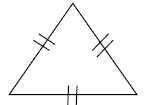• a) 3
• b) 5
• c) 4

#### Question: State the order of rotational symmetry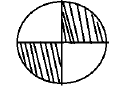• a) 2
• b) 3
• c) 1

#### Question: State the order of rotational symmetry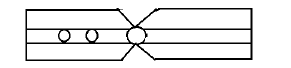• a) 1
• b) 2
• c) 3

#### Question: State the order of rotational symmetry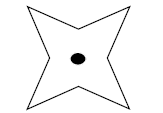• a) 4
• b) 5
• c) 3

#### Question: State the order of rotational symmetry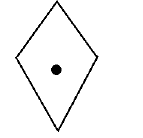• a) 1
• b) 2
• c) 3

#### Question: State the order of rotational symmetry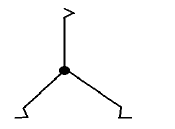• a) 1
• b) 2
• c) 3

#### Question: State the order of rotational symmetry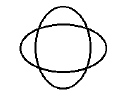• a) 4
• b) 3
• c) 5

• a) G
• b) T
• c) E

#### Question: Which of the following shows reflection symmetry?

• a)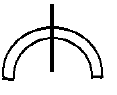• b)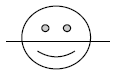• c)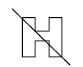#### Question: Which of the following does not have a reflection symmetry?

• a)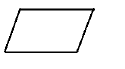• b)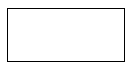• c)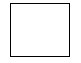#### Question: Which of the following does not have an order 4 of rotational symmetry?

• a)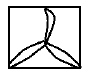• b)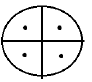• c)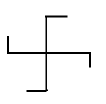#### Question: Which of the following is not symmetrical?

• a)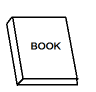• b)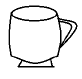• c)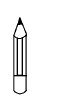#### Question: Choose the correct net for cone?

• a)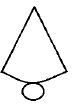• b)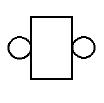• c)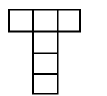#### Question: Which is the correct net for cylinder?

• a)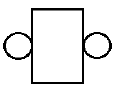• b)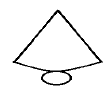• c)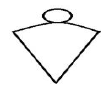#### Question: Which is the correct net for a cube?

• a)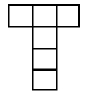• b)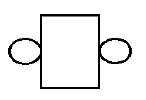• c)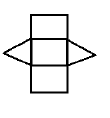• a) cuboid
• b) cone
• c) cube

#### Question: The solid that can be formed from the given net is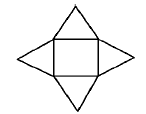• a) square pyramid
• b) triangular prism
• c) cuboid

#### Question: Which of the following is the line of symmetry of the given figure?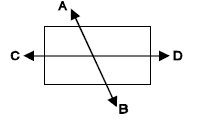• a) CD
• b) none of these
• c) AB

#### Question: Which solid figure has all its faces as triangles?

• a) triangular pyramid
• b) none of these
• c) cone

• a) C
• b) N
• c) H

#### Question: Which of the following set of figures show rotation?

• a)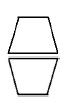• b)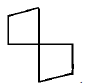• c)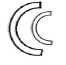• a) A
• b) H
• c) X

#### Question: How many lines of symmetry are there in the following figure?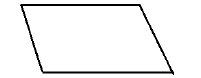• a) 0
• b) 1
• c) 2

#### Question: State the order of rotational symmetry in the following figure.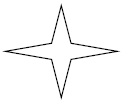• a) 4
• b) 3
• c) 2